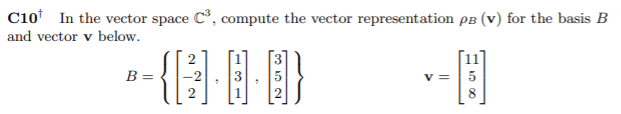## Example

Chapter R: Respresentations

Question C10, Page 512## Solution

To find a solution for:

$\rho_B(y)$

it is necessary to find scalars:

$a_1, a_2, a_3$

such that:

$y = a_1u_1 + a_2u_2 + a_3u_3$

We get the following system of equations:

2a + 1b + 3c = 11

-2a + 3b + 5c = 5

2a + 1b + 2c = 8

We can solve this using matrix row operations and substitution.

A = rbind(c(2,1,3,11),c(-2,3,5,5),c(2,1,2,8))
print(A)
##      [,1] [,2] [,3] [,4]
## [1,]    2    1    3   11
## [2,]   -2    3    5    5
## [3,]    2    1    2    8

First, subtract row 1 from row 3.

A[3,] = A[3,] - A[1,]
print(A)
##      [,1] [,2] [,3] [,4]
## [1,]    2    1    3   11
## [2,]   -2    3    5    5
## [3,]    0    0   -1   -3

From this step, we get that -1c = -3. Solving for c, we get c = 3. We can substitute this into the system of equations and create a new matrix:

2a + 1b + 3(3) = 11

-2a + 3b + 5(3) = 5

The equations above become this:

2a + 1b = 2

-2a + 3b = -10

Adding the two equations together, we get:

4b = -8

b = -2

Finally, solving for a, we get:

2a - 2 = 2

2a = 4

a = 2

Therefore, the representation is:

$\rho_B(y) = \left(\begin{array}{cc} 2\\ -2\\ 3 \end{array}\right)$: